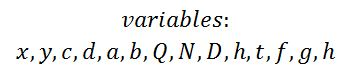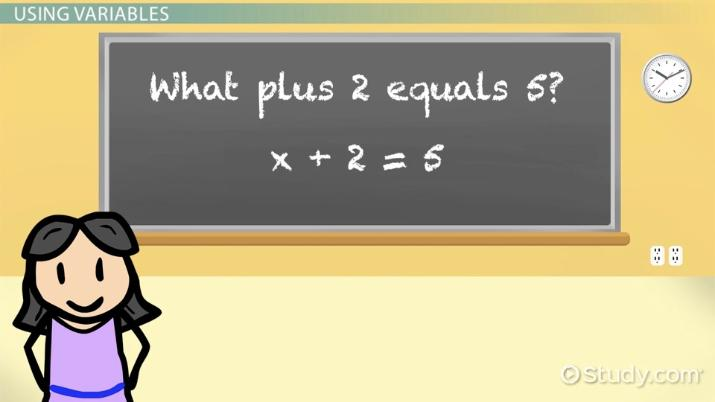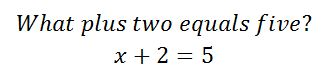Science Courses / Course / Chapter

# Variables in Math

Brigette Banaszak, Yuanxin (Amy) Yang Alcocer
• Author
Brigette Banaszak

Brigette has a BS in Elementary Education and an MS in Gifted and Talented Education, both from the University of Wisconsin. She has taught math in both elementary and middle school, and is certified to teach grades K-8.

• Instructor
Yuanxin (Amy) Yang Alcocer

Amy has a master's degree in secondary education and has been teaching math for over 9 years. Amy has worked with students at all levels from those with special needs to those that are gifted.

What is a variable in math? Learn what a variable is and how to find the variable. See variable examples, their properties, and steps to find their value. Updated: 07/29/2021

Show

## What is a Variable in Math?

In math, a variable is a letter used in place of an unknown number in equations, expressions, and formulas. The variable is used as a placeholder for the unknown number.

In an equation like 11x + 25 = 135, the variable will have a definite, but unknown, value.

In an expression like x - 9, the variable can be replaced with any number to produce a different result.

An example of how variables are used in math is the Pythagorean Theorem:

{eq}a^2 + b^2 = c^2 {/eq}This formula contains three variables, a, b, and c.

Another example of how variables are used in math is the quadratic formula, which is shown here.There are different categories of variables.

• A dependent variable gets its name from the fact that its value depends on something else.
• An independent variable, on the other hand, is not dependent on anything else.
• A free variable is a variable with no limits on its value. A free variable can represent any number. For example, in the function f(x) = 8x, the variable x is a free variable. It can represent any number, positive or negative, whole number, fraction, or decimal.
• A bound variable has parameters that it can not exceed. It is bound within those parameters and can only represent a number within the limitations given.
• A random variable, often used in statistics, is a variable that can take on different values.

In a mathematical function, the independent variable is the input, and the dependent variable is the output. In the equation y = 6x + 9, for example, the variable x is the independent variable. The variable y, on the other hand, is the dependent variable because its value depends on the value of x.

### Key Properties of Variables

What is a variable in math? These are important things to keep in mind when using variables in math:

• A variable in math is a letter. Lowercase letters and uppercase letters are both used.
• Any letter can be used, although x and y are letters used frequently.
• Variables in math are placeholders for unknown numbers.
• In math, variables can be used in expressions, equations, and formulas.
• Variables are often used when the best answer needs to be found.

## Defining Variables

Variables are nothing more than a placeholder. They stand for things that you want to find out but don't have the answer to yet. Think back to grade school when you were learning addition and multiplication. Did your teacher ask you, 'What plus two equals five?' Well, when the teacher uses the word 'what,' she actually used it as a variable. The 'what' is something you wanted to know but didn't have the answer to right away.

Now, of course, you wouldn't even blink at that question. You quickly would answer 'three' and not even think twice about the 'what.'

In math lingo, there is a formality when it comes to variables. When you write them, instead of using the word 'what,' you would use letters. You can use any letter you choose. Typically, we stick to the English alphabet but technically, you can use any easy-to-identify symbol as your choice.

The two most common variables you will encounter in your schooling career are the variables x and y. Don't ask the reason why these two are the most popular. They just are and have been in use for so many years past. They are easy to spot and to identify, which makes them ideal candidates for a wide variety of mathematical applications.

Just because x and y are the two most popular variables doesn't mean you have to use just those two. You can use anything you wish. You have numerous choices to pick from.Any letter you can think of can be used as a variable. They can be uppercase or lowercase. When you get into more complex math applications, you might have several variables in one equation, so your ability to use more than one variable at a time will become important.An error occurred trying to load this video.

Try refreshing the page, or contact customer support.

Coming up next: Double-Digit Addition Without Regrouping

### You're on a roll. Keep up the good work!

Replay
Your next lesson will play in 10 seconds
• 0:05 Defining Variables
• 1:42 Using Variables
• 2:54 When to Use Variables
• 3:42 Lesson Summary
Save Save

Want to watch this again later?

Timeline
Autoplay
Autoplay
Speed Speed

## How to Find the Variable

Sometimes, in a math equation, finding the value of the variable is the goal. In this case, the variable is being used when the best answer needs to be found. To find the value of the variable, the equation can be manipulated.

When an equation is manipulated, it is very important to perform the same operation on both sides of the equation.

In order to keep it a true equation, the two sides must be equal. This means whatever is done to one side of the equation, the same thing must be done to the other side of the equation.

Here is an example of how to find the value of the variable in a math equation:

• Equation: 5x + 8 = 23
• Subtract 8 from both sides of the equation: 5x + 8 - 8 = 23 - 8
• Simplify: 5x = 15
• Divide by 5 on both sides of the equation: 5x / 5 = 15 / 5
• Simplify: x = 3

The equation was manipulated to isolate the variable, x, and find its value.

In this example, the value of the variable is 3.

Some examples require the equation to be manipulated more than others, but the basic steps to follow are always the same.

## Using Variables

Variables have always stood in the place of an unknown answer. Their use remains the same. They always stand for something you want to know but don't have the answer to. With the aid of variables and algebra, you can find out the answer.

Going back to grade school again, let's look more carefully at the question 'What plus two equals five?' We now know that the 'what' stands for a variable. We also know now that we can choose any letter for our variable. So, let us choose x. Why not? It's an easy letter to choose and there is a phrase that goes, 'X marks the spot.' That's easy to remember.To unlock this lesson you must be a Study.com Member.

Video Transcript

## Defining Variables

Variables are nothing more than a placeholder. They stand for things that you want to find out but don't have the answer to yet. Think back to grade school when you were learning addition and multiplication. Did your teacher ask you, 'What plus two equals five?' Well, when the teacher uses the word 'what,' she actually used it as a variable. The 'what' is something you wanted to know but didn't have the answer to right away.

Now, of course, you wouldn't even blink at that question. You quickly would answer 'three' and not even think twice about the 'what.'

In math lingo, there is a formality when it comes to variables. When you write them, instead of using the word 'what,' you would use letters. You can use any letter you choose. Typically, we stick to the English alphabet but technically, you can use any easy-to-identify symbol as your choice.

The two most common variables you will encounter in your schooling career are the variables x and y. Don't ask the reason why these two are the most popular. They just are and have been in use for so many years past. They are easy to spot and to identify, which makes them ideal candidates for a wide variety of mathematical applications.

Just because x and y are the two most popular variables doesn't mean you have to use just those two. You can use anything you wish. You have numerous choices to pick from.Any letter you can think of can be used as a variable. They can be uppercase or lowercase. When you get into more complex math applications, you might have several variables in one equation, so your ability to use more than one variable at a time will become important.

## Using Variables

Variables have always stood in the place of an unknown answer. Their use remains the same. They always stand for something you want to know but don't have the answer to. With the aid of variables and algebra, you can find out the answer.

Going back to grade school again, let's look more carefully at the question 'What plus two equals five?' We now know that the 'what' stands for a variable. We also know now that we can choose any letter for our variable. So, let us choose x. Why not? It's an easy letter to choose and there is a phrase that goes, 'X marks the spot.' That's easy to remember.To unlock this lesson you must be a Study.com Member.

#### Which is an example of variable terms?

A variable is a letter, either lowercase or uppercase. For example, in the equation x + 2 = 5, the x is being used as a variable.

#### How do you define a variable?

In math, a variable is a letter used in place of an unknown number in equations, expressions, and formulas. The variable is used as a placeholder for the unknown number. Any letter can be used as a variable.

### Register to view this lesson

Are you a student or a teacher?

Back

### Resources created by teachers for teachers

Over 30,000 video lessons & teaching resources‐all in one place.Video lessonsQuizzes & WorksheetsClassroom IntegrationLesson Plans

I would definitely recommend Study.com to my colleagues. It’s like a teacher waved a magic wand and did the work for me. I feel like it’s a lifeline.

Jennifer B.
TeacherCreate an account to start this course today
Used by over 30 million students worldwide